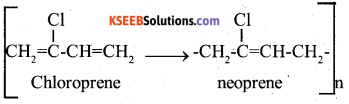# 2nd PUC Chemistry Model Question Paper 3 with Answers

Students can Download 2nd PUC Chemistry Model Question Paper 3 with Answers, Karnataka 2nd PUC Chemistry Model Question Papers with Answers helps you to revise the complete Karnataka State Board Syllabus and score more marks in your examinations.

## Karnataka 2nd PUC Chemistry Model Question Paper 3 with Answers

Time: 3 Hrs 15 Min
Max. Marks: 70

Part-A

I. Answer all of the following. (Each question carries 1 mark) (10 × 1=10)

Question 1.
On what factor the value of colligative property depends?
Number of Solute Particle.

Question 2.
Give an example for liquid solution in which solute is gas.
Soda water.

Question 3.
How many Coulombs of electricity required to oxidise one mole of Al to Al3+?
3 × 96500

Question 4.
In a zero order reaction, the time taken to reduce the concentration of reactant from 50% to 25% is 30 minutes. What is the time required to reduce the concentration from 25% to 12.5%?
15mts.Question 5.
Name the enzyme used in the inversion of cane sugar.
Invertase

Question 6.
Which metal is refined by Van-Arkel method?
Zirconium

Question 7.
Name the noble gas which does not have general noble gas electronic configuration ns2np6.
Helium or He

Question 8.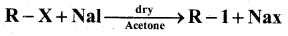What is the name of reaction?
Finkelstein’s reaction.

Question 9.
Which oxidising agent used in Etard – reaction?
CrO2 Cl2 or Chromyl Chloride.

Question 10.
Write the general structure of Zwitter ion.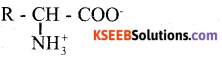Part-B

II. Answer any FIVE of the following. (Each question carries 2 marks) (5 × 2 = 10)

Question 11.
Give any two differences between Frenkel and Schottky defects.
Frenkel defects

1. No change in density
2. Observed when cations and anions dif¬fer in their sizes.

Schottky defects

1. Density decreases
2. Observed when cations and anions have similar sizes

Question 12.
State :
1. Kohlrausch’s law
The limiting molar conductivity of an elec¬trolyte can be represented as the sum of the limiting molar conductivities of the individual cation and anion.

Application: Kohlrausch law is applied to calculate the limiting molar conductivity of any electrolyte from the limiting molar conductivities λ0 of individual ions.

2. Faraday first law of electrolysis
Faraday’s I – law states that “The amount of chemical reaction which occurs at any electrode during electrolysis by a current is directly proportional to the quantity of electricity passed through the electrolyte.Question 13.
Calculate the half-life period of a first order reaction, if the rate constant of the reaction is 6.93 × 10-3 S-1.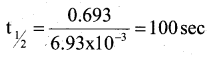Question 14.
Give reasons:
i) Actinoids show variable oxidation states.
ii) Cerium (Ce) exhibits + 4 oxidation state.
Gets octet configuration

Question 15.
a) Write the IUPAC name of major product formed in Friedel – Crafts acylation of anisole.
4 Methoxy acetophenone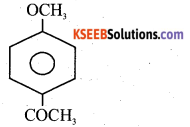b) Complete the reaction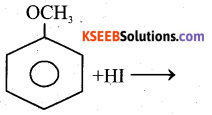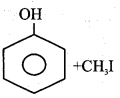Question 16.
How are carboxylic acid prepared from Grignard reagent?
Carbon dioxide gas is bubbled through ethereal solution of Grignard reagent to give an addition compound which on hydrolysis gives carboxylic acid.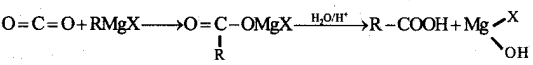Question 17.
What are antibiotics? Give an example.
The chemical substances produced or derived from micro organisms which can inhibit the growth or even destroy the micro organisms are called antibiotics. e.g.Penicillin.

Question 18.
What is Saponification? Give the equation to form sodium stearate by this method.?Part-C

III. Answer any FIVE of the following. (Each question carries three marks) (5 × 3 = 15)

Question 19.
Explain the reducing behaviour of carbon in the extraction of iron using Ellingham diagram.
The formation CO from Coke has less ΔG0 than Fe2O3 above 1073k. CO is more stable than Fe2O3. Hence Cole reduces Fe2O3 into molten Fe above 1073k.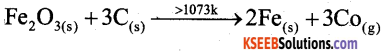Question 20.
a) Name the gas liberated when zinc reacts with dil. HNO3.
N2O

b) Give reason:
1. PH3 has lower boiling point than NH3
Due to Hydrogen bonding

2. Nitrogen is less reactive at room temperature.
Due to half filled stable configuration.

Question 21.
Give the principles involved in the manufacture of sulphuric acid by contact process with equations:
Production of sulphur dioxide: It is carried out by burning powdered sulphur or roasting of sulphur rich ores.
S8 + 8 O2 → 8SO2
4FeS2 +11O2 → 2Fe2O3 + 8SO2

Question 22.
a) Complete the reaction
Cl2 + 2H2O + SO2
H2SO4 + 2HCl

b) i) Which halogen has highest electron affinity or electron gain enthalpy?
Cl > F > Br > I

ii) Give the structure of perchloric acid.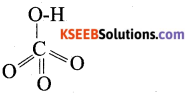Question 23.
a) 3d – series elements exhibit variable oxidation states. Why?

• Due to presence of vacant d-orbitals
• The energy gap 4S and 3d is less

b) Calculate the magnetic moment of Mn2+ ion. [Atomic number of Mn=25].
Mn2+ ion has d5 system, i.e., there are five impaired electrons.Question 24.
Describe the manufacture of potassium dichromate from chromite ore.
i) Preparation of K2Cr2O7 from chromite ore
a) Potassium dichromate is obtained by the fusion of chromite ore (FeCr2O4) with sodium (or potassium) carbonate in the presence of excess of air.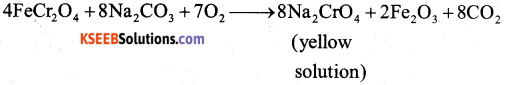b) The solution is filtered and treated with sulphuric acid.
Na2CrO4 + H2SO4 → Na2Cr2O7 + Na2SO4 + H2O

c) Now sodium dichromate is treated with potassium chloride. As a result, potassium dichromate is produced.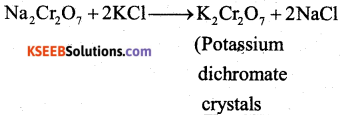ii) Effect of increasing pH on K2Cr2O7 Solution.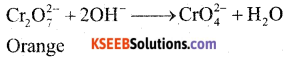On increasing PHI K2Cr2O7 changes into K2CrO4 (orange to yellow)

Question 25.
With the help of Valence Bond Theory (VBT) explain hybridisation, geometry and magnetic property of [CoF6]3- hexa fluoridocobaltate (III) ion.

• sp3d2
• Octahedral
• Paramagnetic ,
[Atomic number of Co = 27]

Question 26.
a) What is the IUPAC name of [Cr(NH3)2 (H2O)3] Cl3?
Triaquatriammine Chromium (iii) Chloride.

b) Give the faical (fac) and meridional (mer) isomeric structures of [Co(NH3)3 (NO2)3]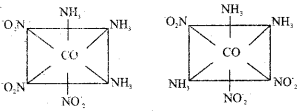Part-D

IV. Answer Any THREE of the following. Each question carries 5 marks. (3 × 5 = 15)

Question 27.
a) Calculate the packing efficiency in Body Centred Cubic (BCC) lattice.
In ΔABG, b2 = a2 + a2
∴ b2 = 2a2;
In, ΔAGD,
C2 = a2 + b2 = a2 + 2a2 ∴ C = $$\sqrt{3 \mathrm{a}}$$
Radius of the atom = r.
Length of the body diagonal C = 4r
$$\sqrt{3 \mathrm{a}}$$ = 4r ; a = $$\frac{4 r}{\sqrt{3}}$$
Edge length of the cube = a = $$\frac{4 r}{\sqrt{3}}$$
Volume of the cubic unit cell = a3 = $$\left(\frac{4 r}{\sqrt{3}}\right)^{3}$$
Volume of one particle (sphere) = $$\frac { 4 }{ 3 }$$ πr3
The number of particles per unit cell of a BCC = 2
Total volume occupied by two spheres = 2 × $$\frac { 4 }{ 3 }$$ πr3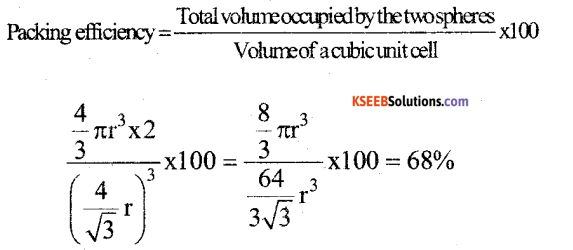b) An element having atomic mass 107.9 gmol-1 has fee unit cell. The edge length of unit cell is 4.8,6 pm. Calculate the density of the unit cell.
[Given NA = 6.002 x 1023 mol-1].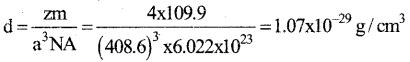Question 28.
a) The boiling point of benzene is 353.23 K when 1.80 g of a non-volatile, non-ionising solute was dissolved in 90 g of benzene, the boiling point is raised to 354.11 K. Calculate the molar mass of the solute.
ΔTb = 354.11K – 353.23K = 0.88K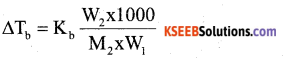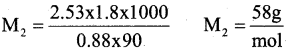b) Write two differences between ideal and non-ideal solutions.
Ideal and Non – Ideal Solutions:

1. There is no interaction between the components in ideal solutions. Where as in non-ideal solutions there is interaction between components.
2. Ideal solution obey’s Raoult’s law whereas non-ideal solution donot obey Raoult’s law.
3. No volume change occurs on mixing due to no interaction in ideal solution whereas in non-ideal solution volume change occurs,Question 29.
a) Draw the neat labelled diagram of Standard Hydrogen Electrode (SHE) and write its symbolic representation.
1. A rectangular platinum plate coated with platinum black is welded to a platinum wire and the wire is fused into a glass tube with the help of mercury.
2. This tube is sealed into a jacket having a side tube at the top. It acts as the hydrogen inlet.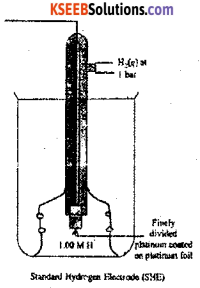3. Two holes are provided at the bottom of the outer jacket, which act as the hydrogen outlet.
4. The outer jacket is immersed in a glass vessel containing 1MHCl.

working: Hydrogen gas is passed from the top inlet. The pressure inside the jacket increases. Due to this, acid level is pushed down and the platinum plate gets exposed to hydrogen gas. Platinum adsorbs hydrogen. As a result, pressure falls and acid level increases back. Now, the adsorbed film of hydrogen comes in contact with hydrogen ions of the solution and equilibrium is established and potential is developed. The potential developed is taken as

Representation of Hydrogen electrode :
H2(g) (1atm) Pt || H+ (1M)

b) Calculate the emf of the cell in which the following reaction takes place
Nis + 2Ag +(0.002m) → Ni2+(0.160M) +2Ag(s)
[Given E0cell = 1.05V]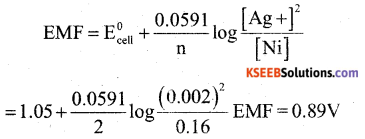Question 30.
a) Derive integrated rate equation for rate constant of zero order reaction.
The reaction which take place in a sequence of elementary reactions to give the products.

b) Show that the rate of first order reaction is doubled when concentration of the reactant is doubled.
A → Products Rate [A]1
When [A] is increases 2 times, rate also increases to two limes.

Question 31.
a) Give any two characteristics of chemisorption.
1. High specificity : Chemisorption is highly specific and it will only occur when adsorbent and adsorbate molecules can chemically react with each other, e.g., oxygen is adsorbed on metals by oxide formation.

2. Surface area: Chemisorption increases with increase with increase in surface area of the adsorbent.

3. What is meant by selectivity of a catalyst?
The catalytic reaction that depends upon the pore structure of the catalyst and the size of the reactant and product molecules is called shape selective catalysis.

b) Define:
1. Brownian movement
The zig-zag movement of colloidal particles is known as Brownian movement.

2. Tyndall effect
When a beam of light is passed through a colloidal solution and the solution is viewed at right angles to the passage of light, they show a mild to strong opalescence, i.e, the path of beam flight is illuminated. This phenomenon is called Tyndall effect. This is cause due to scattering of light by colloidal particles.Part-D

V. Answer any FOUR of the following. (Each question carries 5 marks) (4 x 5 = 20)

Question 32.
a) i) Explain the SN2 mechanism.
When methyl bromide undergoes hydrolysis with aqueous potassium hydroxide, methyl alcohol is formed.
CH3 – Br + KOH → CH3 – OH + KBr
This mechanism involves only one step.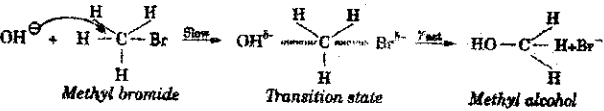The nucleophile OH – attack from the rear side of the leaving group. A transition state with partial formation of C-OH bond and partial breaking of C-Br bond takes place simultaneously.

The rate of the reaction depends both on the concentration of nucleophile as well as alkyl halide. Hence, it is a second order reaction. In SN2 reaction, complete inversion of configuration takes place,

ii) What is the reagent used in the conversion of alkyl halide into alkene?
Alcoholic potassium hydroxide,

b) Complete the reaction.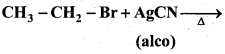When an alkyl halide is heated with alcoholic solution of silver cyanide, alkyl isocyanide is formed. Isocyanides are also called carbylamines.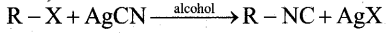Ex: When ethyl bromide is heated with alcoholic silver cyanide solution, ethylisocyanide (ethylcarbylamine) is formed.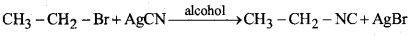c) What are enantiomers?
A pair of optically active isomers which are non-super imposable mirror images of each other.

Question 33.
a) Explain the Kolbe’s reaction.
When sodium phenate is heated with car¬bon dioxide to 140° C under 6-7 atm pressure, sodium salicylate is obtained which on acidification gives salicylic acid.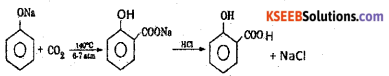b) Complete the reactions: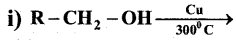R – CHO + H2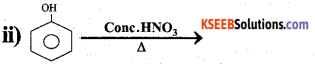When phenol is heated with a mixture of concentrated nitric acid and concentrated sulphuric acid. 2,4,6 – trinitro phenol is obtained called picric acid.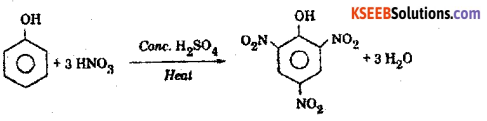c) Write the general equation of Williamson’s ether synthesis.
Alkyl halide react with sodium alkoxide to form ether.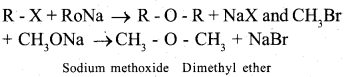Question 34.
a) How would you prepare acetaldehyde from acetyl cholride. Name the reaction.
Aldehyde can be obtained from acid chlorides (RCOCI) by catalystic hydrogentation in presence of palladium catalyst suspended in barium sulphate. This reduction reaction is known as Rosenmund reaction.

b) Name the reagent used in the conversion of ketone to hydrocarbon Name the reaction.
In this reduction reaction the carbonyl group of aldehyde & ketones is reduced to CH2 group on treatment with Zn – Hg and Cone. HCl.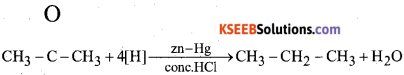c) Acetaldehyde does not undergo Cannizzaro’s reaction. Why?
Acetaldehyde contains three α -hydrogen. Hence it does not answer Cannizzaro’s reaction.

Question 35.
a) Name the major product formed when nitrous acid is treated with
1. methylamine.
Methylamine reacts with nitrous acid to give methyl alcohol liberating nitrogen.
CH3NH2+ONOH → CH3OH+N2+H2O.

2. aniline at low temperature.
In a aniline, the lone pair of electrons present on nitrogen are involved in the delocalisation with n electrons of the benzene ring due to resonance. Hence, the lone pair of electrons present on nitrogen are less available in aniline. In addition, the anilinium ion formed is destabilised by the benzene ring. Hence, aniline is a weaker base than ammonia,

b) Explain the Hoffinann’s bromamide reaction.
When an acid amide is heated with bromine and potassium hydroxide solution, a primary amine is obtained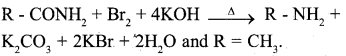c) Write the IUPAC name of (CH3)2N-CH2-CH3
NN1 dimethyl ethanammine

Question 36.
a) Give the Haworth structure of lactose.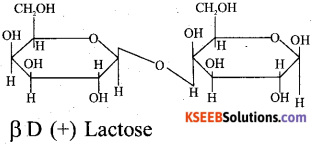b) What are hormones? Give an example.
Hormones are the proteins secreted by ductless glands which regulate growth and reproduction in the body. They are biochemical messengers.

c) Which nitrogenous base present in DNA but not in RNA?
Thymine is a nitrogenous base that is found in DNA but not in RNA. Instead of thymine, RNA molecules utilize uracil.Question 37.
a) How is neoprene prepared?